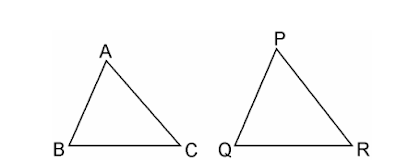Guru

# If the areas of two similar triangles are equal, prove that they are congruent. Q.4

• 0

How i prove the congruent of two similar triangles are equal of exercise 6.4 of class 10th. Sir please help to solve this problem If the areas of two similar triangles are equal, prove that they are congruent.

Share

1. Say ΔABC and ΔPQR are two similar triangles and equal in areaNow let us prove ΔABC ≅ ΔPQR.

Since, ΔABC ~ ΔPQR

∴ Area of (ΔABC)/Area of (ΔPQR) = BC2/QR2

⇒ BC2/QR2 =1 [Since, Area(ΔABC) = (ΔPQR)

⇒ BC2/QR2

⇒ BC = QR

Similarly, we can prove that

AB = PQ and AC = PR

Thus, ΔABC ≅ ΔPQR [SSS criterion of congruence]

• 0